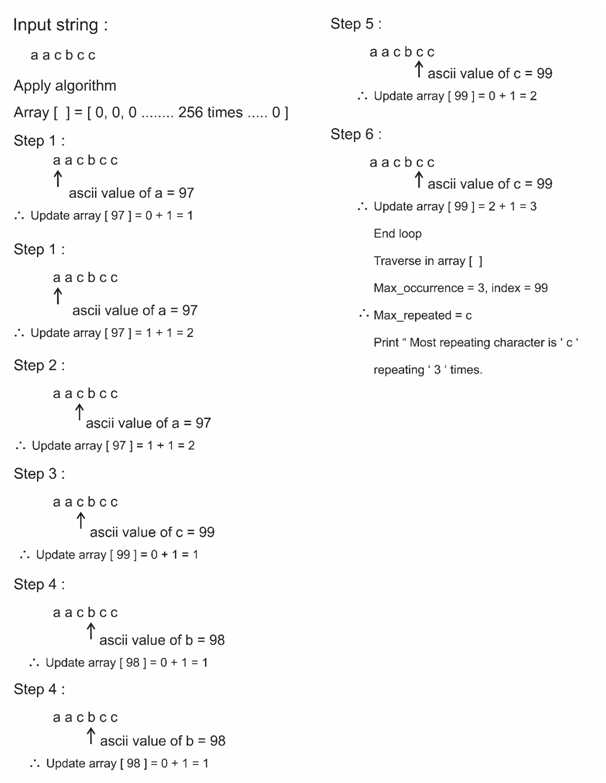# Most repeating character in a string

StringViews 1599

## In the given string find the maximum occurring character

Maximum occurring character: character which is coming more number of times. (Case sensitivity is present, “D” and “d” are not the same.)

Note: If there are more than one character repeated more than once then it prints the first most repeated character.

### Example

a)    Input string = “sachin”

count of ‘s’ = 1
count of ‘a’ = 1
count of ‘c’ = 1
count of ‘h’ = 1
count of ‘i’ = 1
count of ‘n’ = 1

Therefore, Print “No element is repeated”

b)    Input string = “Hello”

count of ‘H’ = 1
count of ‘e’ = 1
count of ‘l’ = 2
count of ‘o’ = 1

Therefore, Print “Most repeated element is l and it occurred 2 times”

c)    Input string = “saAchin”

count of ‘s’ = 1
count of ‘a’ = 1
count of ‘A’ = 1
count of ‘c’ = 1
count of ‘h’ = 1
count of ‘i’ = 1
count of ‘n’ = 1

Therefore, Print “No element is repeated”

d)    Input string = “ccaab”

count of ‘c’ = 2
count of ‘a’ = 2
count of ‘b’ = 1
count of c and a is same but c is coming first so, it prints c.

Therefore, Print “Most repeating element is c”

## Algorithm

Time Complexity : O(n)
Space Complexity : O(1)

Step 1 : Create an array of size 256 with all zeroes. (256 ASCII characters)

Step 2 : Each element in the array is the count of the character with the ASCII value as index in the array. (ASCII value as key)

Step 3 : Increment count whenever we found a character at the index of its ASCII value in the index.

a)    Traverse the whole string.
b)    Update count. If “a” comes array = array + 1 (96 is ASCII value of a)

Step 4 : Initialize max_occurrence= INT_MIN, and find the max_occurrence in the array (max_occurrence comes for character with maximum count) by running a loop and comparing with elements in the array.

Step 5 : Finally print the character with max_occurrence(maximum count)and print the max_occurrence.

### Algorithm working## C++ Program

```#include <bits/stdc++.h>
using namespace std;
#define NO_OF_CHARS 256
int main()
{
string s; //a string
cin>>s;

int arr[NO_OF_CHARS] = {0}; // stores the count of each character in the string

for(int i = 0; i < s.size(); i++) //treat element's ASCII value as key and i?ncrement the count
arr[(int)s[i]]++;

int max_occurence = INT_MIN; //store the maximum occurence of a character
char most_repeated; //final most repeating character

for(int i=0; i<s.size(); i++)
{
if(arr[s[i]] > max_occurence) //update the maximum repeating character
{
most_repeated = s[i];
max_occurence = arr[s[i]];
}
}
if(max_occurence>1){
cout<<"Most repeating character is "<<" ' "<<most_repeated<<" ' "<<" repeating " <<max_occurence<<" times\n";
return 0;
}
else if(max_occurence = 1)
{
cout<<"No character is repeated\n";
}
}```

Translate »